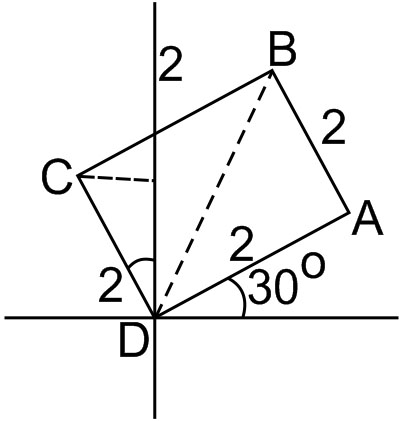#### A square, of each side 2, lies above the x-axis and has one vertex at the origin.  If one of the sides passing through the origin makes an angle 300 with the positive direction of the x-axis, then the sum of the x-coordinates of the vertices of the square Option 1)Option 2)Option 3)Option 4)As we learnt in

Parametric form -- wherein

Whereis the inclination of the line andis the distance betweenandx-coordinates of A isangle of OB with positive x-axis isHence x - coordinate of B isx-cordinate of C is -2sin=-1

Hence Sum=Option 1)This option is correct

Option 2)This option is incorrect

Option 3)This option is incorrect

Option 4)This option is incorrect

#### Plabita